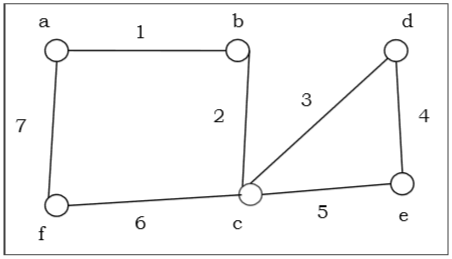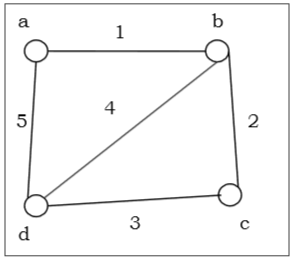# Eulerian Graphs

Euler Graph - A connected graph G is called an Euler graph, if there is a closed trail which includes every edge of the graph G.

Euler Path - An Euler path is a path that uses every edge of a graph exactly once. An Euler path starts and ends at different vertices.

Euler Circuit - An Euler circuit is a circuit that uses every edge of a graph exactly once. An Euler circuit always starts and ends at the same vertex. A connected graph G is an Euler graph if and only if all vertices of G are of even degree, and a connected graph G is Eulerian if and only if its edge set can be decomposed into cycles.The above graph is an Euler graph as a 1 b 2 c 3 d 4 e 5 c 6 f 7 g covers all the edges of the graph.

## Non-Euler GraphHere degree of vertex b and d is 3, an odd degree and violating the euler graph condition.